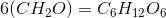# AP Chemistry : Organic Compounds

## Example Questions

### Example Question #42 : Compounds And Molecules

Which of the following is the alcohol functional group?

-OR

-COOH

-COH

-NH2

-OH

-OH

Explanation:

The alcohol functional group is -OH

### Example Question #43 : Compounds And Molecules

What is the most acidic functional group on the amino acid cysteine? Cysteine has the chemical formula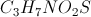.

Thiol

Carboxylic acid

Hydrochloric acid

Amine

Alcohol

Carboxylic acid

Explanation:

We know that by definition, all amino acids contain a carboxylic acid functional group (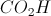), and an amino functional group (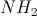). Therefore the additional functional group can only contain sulfur and hydrogen.

The amino functional group is slightly basic, and the carboylic acid functional group is slightly acidic. The -SH functional group will also be acidic, but because sulfur and oxygen are in the same group on the periodic table, the -SH group will have similar acidic properties to an -OH group, and alcohols are less acidic than carboxylic acids.

### Example Question #44 : Compounds And Molecules

Which of the following functional groups is an amide?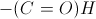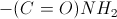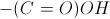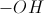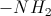Explanation:

Amides contain both oxygen and nitrogen in the functional group. The oxygen forms a double bond with a carbon, and the carbon bonds to a nitrogen. This forms the structure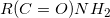, where the nitrogen is also bound to the carbon.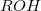is an alcohol functional group.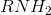is an amine functional group.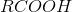is a carboxylic acid functional group.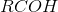is an aldehyde functional group.

### Example Question #45 : Compounds And Molecules

Which one of the following organic functional groups does not contain a carbon-oxygen double bond?

Nitrile

Ester

Aldehyde

Carboxylic acid

Ketone

Nitrile

Explanation:

A nitrile group contains a carbon-nitrogen triple bond, while all of the other groups contain a carbon-oxygen double bond.

Nitrile: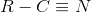Ester: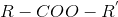Ketone: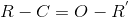Carboxylic acid: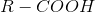Aldehyde: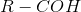### Example Question #46 : Compounds And Molecules

Which of the following is an organic compound?

Sodium chloride

Glucose

Oxygen

Water

Magnesium fluoride

Glucose

Explanation:

An organic compound is typically defined as one that contains carbon. Though there some small exceptions to this rule (for example, cyanide is not considered organic), in general most compounds follow it.

Though water, oxygen, and sodium chloride, may seem organic, they are not for they do not contain any covalent bonds to carbon. The only gievn compound that contains carbon is glucose, a sugar.

### Example Question #47 : Compounds And Molecules

A chemist is trying to find the identity of a compound. She knows that the compound has a molecular weight of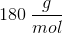, and also that it consists ofcarbon,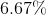hydrogen, and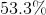oxygen by mass. What are the empirical and molecular formulas, respectively, for this compound?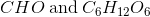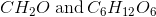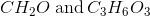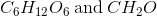Explanation:

The simplest way to begin these types of problems is to assume that we are starting out with 100 grams of the sample compound. This allows us to simplify things, since we know the mass percentages of the elements that make up out unknown compound.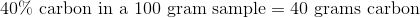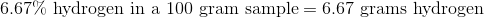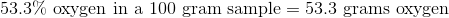Next, we must convert amount of each element from grams into moles.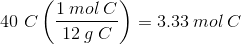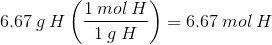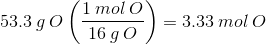This gives us a formula of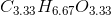Now, we need to simplify this formula by dividing each of the terms by the greatest common denominator, 3.33, which gives us: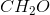This is our empirical formula. To obtain the molecular formula, we must first find the mass of our empirical formula, which is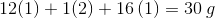.

Next, we need to divide the molecular weight of the compound by the empirical weight to obtain an integer of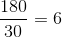. Finally, we multiply the empirical formula by this integer in order to obtain the molecular formula, which is: